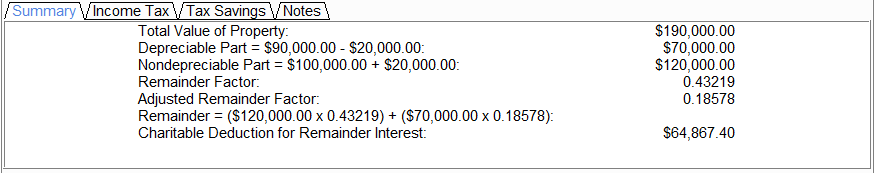Forums Support Library CFP – Charitable Financial Planner Charitable Remainder in Residence/Farm Summary Report

Tagged: ,

• Author
Posts
•For a TERM calculation
The summary report shows the direct results from the values that are entered into the input fields.

• Total Value of Property: This number is the sum of the Land Value and the Depreciable Property Value on the Date of Gift.
• Depreciable Part (DP): Indicates the dollar value of the property that is depreciable. Use the following formula to attain the value:
• (DP Value On Date of Gift – DP value At End of Useful Life) x (Payment Years or DP Value At End of Useful Life* / DP Value At End of Useful Life)
• *Use the smallest value of the two: Payment Years or DP Value At the End of Useful Life.
• Nondepreciable Part: Indicates the dollar value of the property that does not depreciate in value. Use the following formula to attain the value:
• (Land Value – DP Value At End of Useful Life)
• Remainder Factor: This factor is used to calculate the charitable deduction. The program derives this number from the Calculation Type used and the ages entered for each life (if a Life calculation is used.).
• Remainder Interest: This factor is used to calculate the charitable deduction. The program derives this number from the Estimated Useful Life in Years input (1-99 years), the number of lives used and the ages entered for each life. When valuing the remainder interest, if part of the real property is depreciable, the adjusted value is subtracted from the value of the depreciable part.
• Charitable Deduction for Remainder Interest: This is the charitable deduction that is derived from the following formula:
• (Nondepreciable Part x Remainder Factor) + (Depreciable Part x Adjusted Remainder Factor)

For a LIFE calculation
The summary tab shows the direct results from the values that are entered into the input fields.

• Total Value of Property: This number is the sum of the Land Value and the Depreciable Property Value on the Date of Gift.
• Depreciable Part: This number represents the dollar value of the part of the property which depreciates over time. The result is obtained from the following equation:
• Depreciable Amount on Date of Gift – Depreciable Amount at End of Useful Life
• Non-depreciable Part: This number represents the dollar value of the part of the property which does not depreciate over time. The result is obtained from the following equation:
• Land Value + Depreciable Amount at End of Useful Life
• Remainder Factor: This number can be obtained from column 4 of Table A for transfer dates prior to May 1989. Use column 4 of Table S (this value can be obtained from the IRS Publication 1457, Actuarial Values Alpha Volume) for transfer dates after April 1989. It is applied to the non-depreciable part of the property.
• Adjustment Factor: The adjustment factor is determined by dividing (i) by (ii):

(i) s the difference between:

A. the R-factor in column 2 of Table C opposite the initial age of the life tenant in column 1.
B. the R-factor in column 2 of such Table opposite the terminal age in column 1.

(ii) is the product of:

• the estimated useful life of the depreciable property.
• the D-factor in column 3 of the Table opposite the initial age of the life tenant in column 1.

This adjustment factor is carried to five decimal places. Different R-factors and D-factors are used for transfers after April 1989, as opposed to those used for transfers prior to May 1989.

• Charitable Deduction for Remainder Interest: Valuation of a remainder interest in real property following one life is determined under IRS rules (§25.2512-5(d) for transfers after November 30, 1983). The remainder interest is obtained from the following formula:

(Nondepreciable Part x Remainder Factor) + (Depreciable Part x Adjusted Remainder Factor)